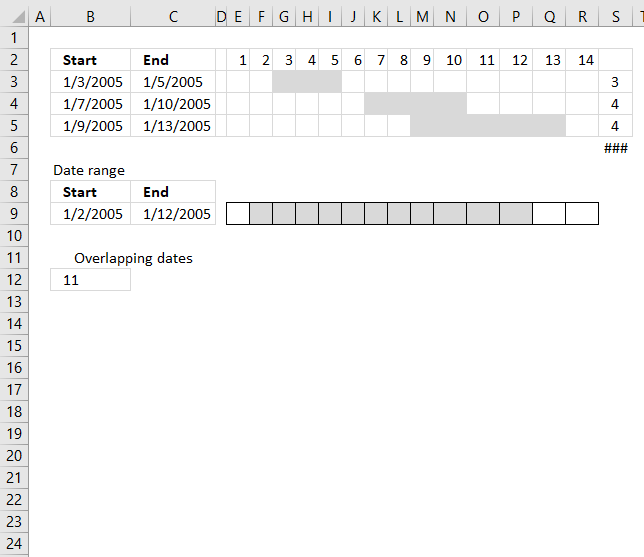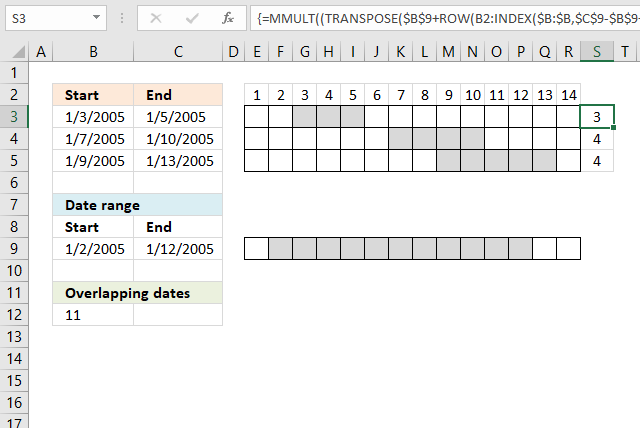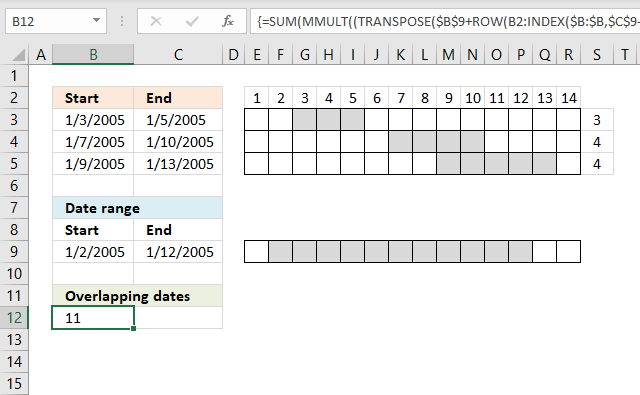Author: Oscar Cronquist Article last updated on April 29, 2021The MEDIAN function lets you count overlapping dates between two date ranges. If you have more than two date ranges you need to use a more complicated array formula.

## 1. Count overlapping days for all date rangesI have three date ranges (B3:C5) in this example and I want to count the number of days that overlap another date range (B9:C9).

This array formula counts overlapping days for each date range in cell range B3:C5 compared to the date range in cell range B9:C9.

Array formula in cell S3:S5:

=MMULT((TRANSPOSE(\$B\$9+ROW(A1:INDEX(\$A:\$A,\$C\$9-\$B\$9+1))-1)>=\$B\$3:\$B\$5)*(TRANSPOSE(\$B\$9+ROW(A1:INDEX(\$A:\$A,\$C\$9-\$B\$9+1))-1)<=C3:C5),ROW(A1:INDEX(\$A:\$A,\$C\$9-\$B\$9+1))^0)

Excel 365 formula in cell R2:

=LET(y, SEQUENCE(B8-A8+1), x, TRANSPOSE(A8+y-1), MMULT((x>=A2:A4)*(x<=B2:B4), y^0))

The Excel 365 formula above is a dynamic array formula and works only in Excel 365.

Both formulas above return this array: {3; 4; 1}, the numbers correspond to the date ranges in rows 2,3, and 4.

Date range 2005-01-03/2005-01-05 (A2:B2) has 3 overlapping dates compared to 2005-01-02/2005-01-12 (A8:B8).

Date range 2005-01-07/2005-01-10 (A3:B3) has 4 overlapping dates compared to 2005-01-02/2005-01-12 (A8:B8).

Date range 2005-01-12/2005-01-13 (A3:B3) has 1 overlapping date compared to 2005-01-02/2005-01-12 (A8:B8).

### Explaining formula in cell S3:S5

\$C\$9-\$B\$9+1

becomes

38364-38354+1

and returns 11.

#### Step 2 - Create a cell reference

The INDEX function is also able to create a cell reference.

INDEX(\$B:\$B, \$C\$9-\$B\$9+1)

becomes

INDEX(\$B:\$B, 11)

and returns A11.

#### Step 3 - Concatenate cell references

A1:INDEX(\$A:\$A, \$C\$9-\$B\$9+1)

returns A1:A11.

#### Step 4 - Calculate row numbers based on cell reference

The ROW function returns a row number from a cell reference. It returns multiple row numbers if the cell reference points to a cell range.

ROW(A1:INDEX(\$A:\$A, \$C\$9-\$B\$9+1))

becomes

ROW(A1:A11)-1

and returns {1; 2; 3; 4; 5; 6; 7; 8; 9; 10; 11}

#### Step 5 - Subtract with 1

ROW(A1:INDEX(\$A:\$A, \$C\$9-\$B\$9+1))-1

becomes

{1; 2; 3; 4; 5; 6; 7; 8; 9; 10; 11} - 1

and returns

{0; 1; 2; 3; 4; 5; 6; 7; 8; 9; 10}

#### Step 6 - Add array to start date

\$B\$9+ROW(A1:INDEX(\$A:\$A, \$C\$9-\$B\$9+1))-1

becomes

38354+{0; 1; 2; 3; 4; 5; 6; 7; 8; 9; 10}

and returns {38354; 38355; 38356; 38357; 38358; 38359; 38360; 38361; 38362; 38363; 38364}

#### Step 7 - Convert vertical array to a horizontal array

The TRANSPOSE function allows you to convert a vertical range to a horizontal range, or vice versa.

TRANSPOSE(array)

TRANSPOSE(\$B\$9+ROW(A1:INDEX(\$A:\$A, \$C\$9-\$B\$9+1))-1)

becomes

TRANSPOSE({38354; 38355; 38356; 38357; 38358; 38359; 38360; 38361; 38362; 38363; 38364})

and returns

{38354, 38355, 38356, 38357, 38358, 38359, 38360, 38361, 38362, 38363, 38364}

#### Step 8 - Test which dates are larger or equal to start dates

TRANSPOSE(\$B\$9+ROW(A1:INDEX(\$A:\$A,\$C\$9-\$B\$9+1))-1)>=\$B\$3:\$B\$5

becomes

{38354, 38355, 38356, 38357, 38358, 38359, 38360, 38361, 38362, 38363, 38364}>=\$B\$3:\$B\$5

becomes

{38354, 38355, 38356, 38357, 38358, 38359, 38360, 38361, 38362, 38363, 38364}>={38355; 38359; 38361}

and returns

{FALSE, TRUE, TRUE, TRUE, TRUE, TRUE, TRUE, TRUE, TRUE, TRUE, TRUE;FALSE, FALSE, FALSE, FALSE, FALSE, TRUE, TRUE, TRUE, TRUE, TRUE, TRUE;FALSE, FALSE, FALSE, FALSE, FALSE, FALSE, FALSE, TRUE, TRUE, TRUE, TRUE}

#### Step 9 - Test which dates are smaller or equal to end dates

TRANSPOSE(\$B\$9+ROW(A1:INDEX(\$A:\$A,\$C\$9-\$B\$9+1))-1)<=C3:C5

becomes

{38354, 38355, 38356, 38357, 38358, 38359, 38360, 38361, 38362, 38363, 38364}<=C3:C5

becomes

{38354, 38355, 38356, 38357, 38358, 38359, 38360, 38361, 38362, 38363, 38364}<={38357;38362;38365}

and returns

{TRUE, TRUE, TRUE, TRUE, FALSE, FALSE, FALSE, FALSE, FALSE, FALSE, FALSE;TRUE, TRUE, TRUE, TRUE, TRUE, TRUE, TRUE, TRUE, TRUE, FALSE, FALSE;TRUE, TRUE, TRUE, TRUE, TRUE, TRUE, TRUE, TRUE, TRUE, TRUE, TRUE}

#### Step 10 - Multiply arrays

This step identifies dates inside a date range, both arrays must return TRUE to return TRUE which is the same as AND-logic.

(TRANSPOSE(\$B\$9+ROW(A1:INDEX(\$A:\$A,\$C\$9-\$B\$9+1))-1)>=\$B\$3:\$B\$5)*(TRANSPOSE(\$B\$9+ROW(A1:INDEX(\$A:\$A,\$C\$9-\$B\$9+1))-1)<=C3:C5)

becomes

{FALSE, TRUE, TRUE, TRUE, TRUE, TRUE, TRUE, TRUE, TRUE, TRUE, TRUE;FALSE, FALSE, FALSE, FALSE, FALSE, TRUE, TRUE, TRUE, TRUE, TRUE, TRUE;FALSE, FALSE, FALSE, FALSE, FALSE, FALSE, FALSE, TRUE, TRUE, TRUE, TRUE}*{TRUE, TRUE, TRUE, TRUE, FALSE, FALSE, FALSE, FALSE, FALSE, FALSE, FALSE;TRUE, TRUE, TRUE, TRUE, TRUE, TRUE, TRUE, TRUE, TRUE, FALSE, FALSE;TRUE, TRUE, TRUE, TRUE, TRUE, TRUE, TRUE, TRUE, TRUE, TRUE, TRUE}

and returns

{0, 1, 1, 1, 0, 0, 0, 0, 0, 0, 0;0, 0, 0, 0, 0, 1, 1, 1, 1, 0, 0;0, 0, 0, 0, 0, 0, 0, 1, 1, 1, 1}.

Note that 1 is equal to the boolean value TRUE and 0 (zero) is FALSE.

#### Step 11 - Create an array of numbers all equal to 1

Exponentiation is a mathematical operation, use the ^ character or the POWER function to calculate the result of exponentiation.

When a number is raised to the power of 0 (zero) the result is always 1. This makes it easy to create an array of numbers all equal to 1.

ROW(A1:INDEX(\$A:\$A,\$C\$9-\$B\$9+1))^0

becomes

{1;2;3;4;5;6;7;8;9;10;11}^0

and returns

{1; 1; 1; 1; 1; 1; 1; 1; 1; 1; 1}

#### Step 12 - Evaluate MMULT function

The MMULT function calculates the matrix product of two arrays, an array as the same number of rows as array1 and columns as array2.

MMULT((TRANSPOSE(\$B\$9+ROW(A1:INDEX(\$A:\$A,\$C\$9-\$B\$9+1))-1)>=\$B\$3:\$B\$5)*(TRANSPOSE(\$B\$9+ROW(A1:INDEX(\$A:\$A,\$C\$9-\$B\$9+1))-1)<=C3:C5),ROW(A1:INDEX(\$A:\$A,\$C\$9-\$B\$9+1))^0)

becomes

MMULT({0, 1, 1, 1, 0, 0, 0, 0, 0, 0, 0;0, 0, 0, 0, 0, 1, 1, 1, 1, 0, 0;0, 0, 0, 0, 0, 0, 0, 1, 1, 1, 1},{1; 1; 1; 1; 1; 1; 1; 1; 1; 1; 1})

and returns {3;4;4}.

## 2. Count all overlapping daysTo count all overlapping days, array formula in cell B12:

=SUM(MMULT((TRANSPOSE(\$A\$8+ROW(A1:INDEX(\$A:\$A, \$B\$8-\$A\$8+1))-1)>=\$A\$2:\$A\$4)*(TRANSPOSE(\$A\$8+ROW(A1:INDEX(\$A:\$A, \$B\$8-\$A\$8+1))-1)<=B2:B4), ROW(A1:INDEX(\$A:\$A, \$B\$8-\$A\$8+1))^0))

Functions in array formulas: MMULT, ROW, INDEX

### Overlapping date ranges in cell range A2:B4

Keep in mind that if you have overlapping date ranges in A2:B4, overlapping dates will be counted twice or more.

In this example, date 9 and 10 are overlapped by 2005-01-07/2005-01-10 and 2005-01-09/2005-01-13. They are counted twice, see values above in cell range S4:S5.

The value in cell A11 is wrong because of this, only 9 dates are overlapped. There is a formula for this scenario also but I'll save it for a future post.